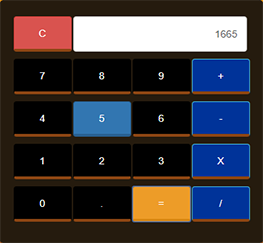# Calculator Using JavaScript

This is developed using HTML, CSS, JavaScript & Bootstrap.
All our calculator will do is basic arithmetic: add, subtract, multiply and divide. It includes a display area of numbers, numeric buttons 0-9, decimal point button, function buttons(+,-,*,/ & C), and Calculate button ( = ).
It is designed simply just like a normal calculator that performs numeric operations so that user can operate it and understand easily

Article Rating
Subscribe
Notify of1 Comment
Inline Feedbacks